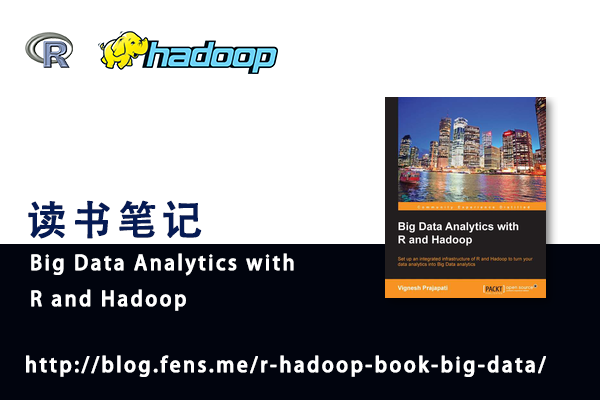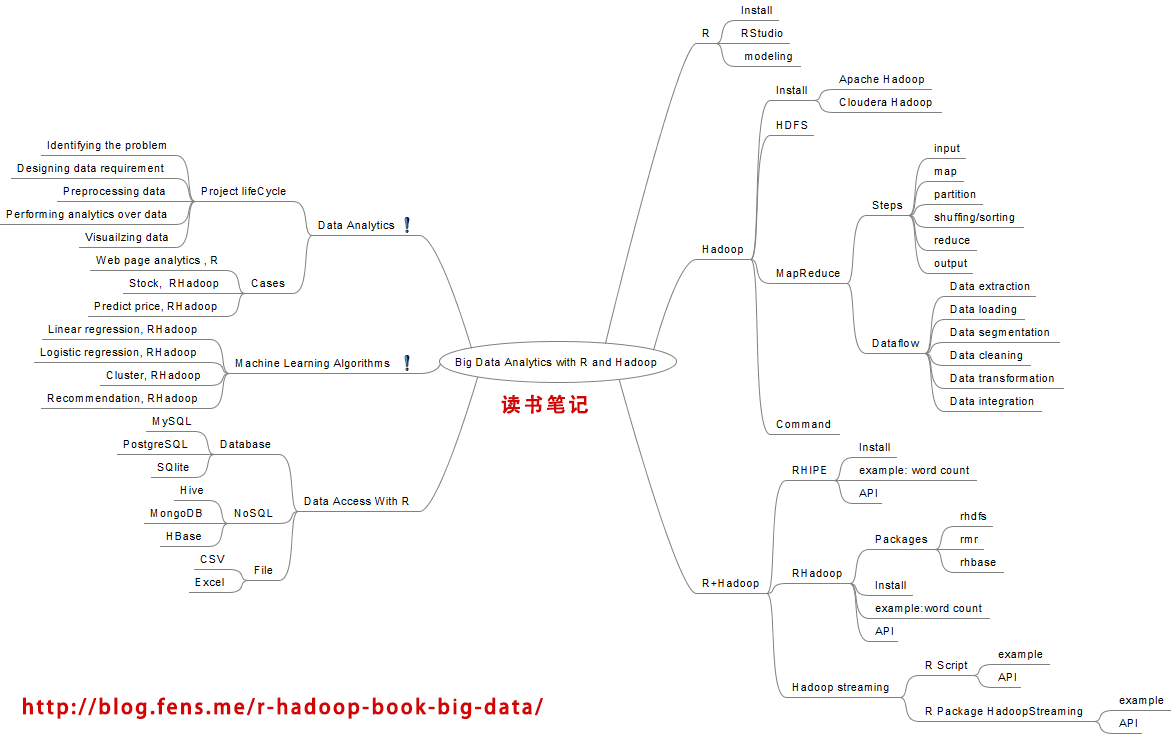• Posts tagged "Big Data Analytics with R and Hadoop"

# Blog Archives

## 读书笔记 Big Data Analytics with R and Hadoop

RHadoop实践系列文章，包含了R语言与Hadoop结合进行海量数据分析。Hadoop主要用来存储海量数据，R语言完成MapReduce 算法，用来替代Java的MapReduce实现。有了RHadoop可以让广大的R语言爱好者，有更强大的工具处理大数据1G, 10G, 100G, TB, PB。 由于大数据所带来的单机性能问题，可能会一去不复返了。

RHadoop实践是一套系列文章，主要包括”Hadoop环境搭建”，”RHadoop安装与使用”，R实现MapReduce的协同过滤算法”，”HBase和rhbase的安装与使用”。对于单独的R语言爱好者，Java爱好者，或者Hadoop爱好者来说，同时具备三种语言知识并不容 易。此文虽为入门文章，但R,Java,Hadoop基础知识还是需要大家提前掌握。

• 张丹(Conan), 程序员Java,R,PHP,Javascript
• weibo：@Conan_Z
• blog: http://blog.fens.me
• email: bsspirit@gmail.com1. 图书概览
2. 图书内容剖析
3. 最后总结

## 1. 图书概览## 2. 图书内容剖析

• R语言介绍
• Hadoop介绍
• R+Hadoop技术方案
• 数据分析案例
• 大数据分析案例
• R语言的数据访问接口

1). R语言介绍

2). Hadoop介绍

3). R+Hadoop技术方案

a. RHipe

RHipe是R与Hadoop的集成编程环境。RHIPE可以让R语言与Hadoop进行通信，访问Hadoop的HDFS和调用MapReduce，让R语言的使用者利用Hadoop的分步式环境进行行大数据的分析。

RHipe官方网站：http://www.datadr.org/

b. RHadoop

RHadoop是由RevolutionAnalytics公司开发的一个R与Hadoop的集成编程环境，与RHipe的功能一样。RHadoop包含三个R包 (rmr，rhdfs，rhbase)，分别是对应Hadoop系统架构中的，MapReduce, HDFS, HBase 三个部分。在2013年底，又增加第4个R包plyrmr，用于数据处理操作。本书中并没有涉及plyrmr包。

RHadoop实践系列文章：http://blog.fens.me/series-rhadoop/

c. R + Hadoop Streaming

Hadoop Streaming是Hadoop提供的，允许任何可执行的脚本作为Mapper和Reducer的一种实现方实。R + Hadoop Streaming就是用R脚本实现Mapper和Reducer。作者用2种方式，进行了测试。

c1. R Script + Hadoop Streaming : 单纯的R语言脚本，通过Shell运行。
c2. HadoopStreaming + Hadoop Streaming: 使用一个封装好的R包HadoopStreaming来实现。

4). 数据分析案例

• 问题
• 定义数据需求
• 数据预处理
• 数据建模及执行
• 数据可视化

• 网页分类：把一个网站中的网页按重要性进行排名
• 股票分析：通过历史交易数据计算股票市场的变化
• 价格预测：预测图书销售的价格，来自Kaggle的一道竞赛题

5). 机器学习算法案例

4个算法的案例：

a. 线性回归

``y = ax + e ``

``````
# Reducer
Sum = function(., YY) keyval(1, list(Reduce('+', YY)))

# XtX =
values(

# For loading hdfs data in to R
from.dfs(

# MapReduce Job to produce XT*X
mapreduce(
input = X.index,

# Mapper – To calculate and emitting XT*X
map =
function(., Xi) {
yi = y[Xi[,1],]
Xi = Xi[,-1]
keyval(1, list(t(Xi) %*% Xi))},

# Reducer – To reduce the Mapper output by performing sum
operation over them
reduce = Sum,
combine = TRUE)))[]
``````

b. Logistic回归

``````
logit(p) = β0 + β1 × x1 + β2 × x2 + ... + βn × xn
``````

R程序还是一个函数glm()。

``````
# Mapper – computes the contribution of a subset of points to the
gradient.

lr.map =
function(., M) {
Y = M[,1]
X = M[,-1]
keyval(1, Y * X * g(-Y * as.numeric(X %*% t(plane))))
}

# Reducer – Perform sum operation over Mapper output.

lr.reduce = function(k, Z) keyval(k, t(as.matrix(apply(Z,2,sum))))

# MapReduce job – Defining MapReduce function for executing logistic
regression

logistic.regression =
function(input, iterations, dims, alpha){
plane = t(rep(0, dims))
g = function(z) 1/(1 + exp(-z))
for (i in 1:iterations) {
gradient =
values(
from.dfs(
mapreduce(input, map = lr.map, reduce = lr.reduce, combine = T)))
plane = plane + alpha * gradient
}
plane
}
``````

c. 聚类算法

``````
# distance calculation function
dist.fun = function(C, P) {
apply(C,1,function(x) colSums((t(P) - x)^2))
}

# k-Means Mapper
kmeans.map = function(., P) {
nearest = {

# First interations- Assign random cluster centers
if(is.null(C))
sample(1:num.clusters,nrow(P),replace = T)

# Rest of the iterations, where the clusters are assigned # based
on the minimum distance from points

else {
D = dist.fun(C, P)
nearest = max.col(-D)}}
if(!(combine || in.memory.combine))
keyval(nearest, P)
else
keyval(nearest, cbind(1, P))
}

# k-Means Reducer
kmeans.reduce = {

# calculating the column average for both of the conditions
if (!(combine || in.memory.combine) )
function(., P) t(as.matrix(apply(P, 2, mean)))
else function(k, P) keyval(k,t(as.matrix(apply(P, 2, sum))))
}

# k-Means MapReduce – for
kmeans.mr = function(P,num.clusters,num.iter,combine,in.memory.combine) {
C = NULL
for(i in 1:num.iter ) {
C =  values(from.dfs(mapreduce(P,map = kmeans.map,reduce = kmeans.reduce)))
if(combine || in.memory.combine)
C = C[, -1]/C[, 1]
if(nrow(C) < num.clusters) {
C =rbind(C,matrix(rnorm((num.clusters -nrow(C)) * nrow(C)),ncol = row(C)) %*% C)
}
}
C
}
``````

d. 推荐算法

``同现矩阵 * 评分矩阵 = 推荐结果``

R语言的算法实现：http://blog.fens.me/r-mahout-usercf/

RHadoop分步式算法实现：http://blog.fens.me/rhadoop-mapreduce-rmr/

6). R语言的数据访问接口

FILE:

• CSV: read.csv(), write.csv()
• Excel: xlsx, xlsxjars,rJava

Database:

• MySQL: RMySQL
• SQLite: RSQLite
• PostgreSQL: RPostgreSQL

NoSQL:

• MongoDB: rmongodb
• Hive: RHive
• HBase: RHBase

R的多种接口已经打通，这也就说明R已经做好了准备，为工业界带来革命的力量。

## 3. 最后总结